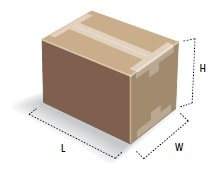of
• UPS is open for business: Service impacts related to Coronavirus...More
Skip To Main Content

# Billable Weight

Billable weight is the weight used to calculate the rate. For domestic and international services, the billable weight will be the greater of the dimensional weight compared to the actual weight.

This document contains:

## Determine the Actual Weight

Actual weight is the package weight rounded up to the next whole pound.

Use a scale to determine the weight of the package. Round any fraction of a pound to the next whole pound for UPS Express® Envelopes (letters) over eight ounces and all other packages.

Back to the Top

## Determine Dimensional Weight

Dimensional weight reflects package density, which is the amount of space a package occupies in relation to its actual weight. Dimensional weight may apply to all UPS domestic and international package services.

Determine the package dimensions in inches. For each dimension, measure at the longest point, rounding each measurement to the nearest whole number (for example, 1.00 to 1.49 will be considered 1, and 1.50 to 1.99 will be considered 2).

Multiply the package length (longest side of the package) by the width by the height.  The result is the cubic size in inches.

Divide the cubic size in inches by the divisor to calculate the dimensional weight in pounds. Increase any fraction to the next whole pound.

The divisor varies by rate type: 139 for Daily Rates; 166 for Retail Rates.

Dimensional Weight = (L x W x H) ÷ Divisor

L = Length in inches

W = Width in inches

H = Height in inches

Divisor for Daily Rates = 139

Divisor for Retail Rates = 166Back to the Top

## Determine Billable Weight

Compare the package's actual weight to its dimensional weight. The greater of the two is the billable weight and should be used to calculate the rate. For multiple-package shipments, total the billable weight of all packages in the shipment.

For additional information, refer to the Determine the Weight and Size section of the Rate and Service Guide.

View Rate and Service Guides

How to Measure Your Package Size

Back to the Top

## Related Information